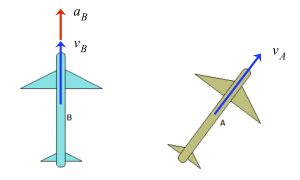# Relative motionAircrafts A and B are flying on the same horizontal plane. Aircraft A is traveling with a constant speed vA, whereas B is traveling with a speed of vB that is increasing at a rate of aB. We are interested in understanding the relative motion between the two aircrafts.

Stationary observer
Shown below is an animation of the motion of A and B as seen by a stationary observer. The paths of A and B are straight, as expected, with the speed of B increasing in time.View of B as seen by an observer on A
Shown below is an animation of the motion of aircraft B as seen by a passenger on aircraft A. The observed path of B is curving off to the left and backward. Can you see this in the relative motion equations of: vB/A = vB - vA?View of A as seen by an observer on B
Shown below is an animation of the motion of aircraft A as seen by a passenger on aircraft B. The observed path of A is curving off to the right and forward. Can you see this in the relative motion equations of: vA/B = vA - vB?NOTE:
In this case, the observed motion is the same as the relative motion, since neither observer is rotating. In the next chapter, we will consider observers on rotating reference frames. In that case, the observed motion becomes much more complex.